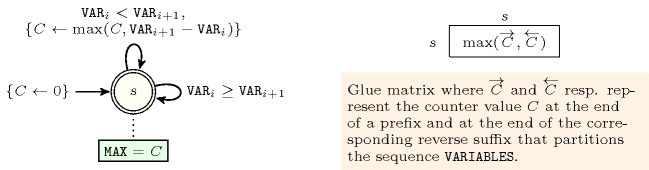## 5.241. max_increasing_slope

Origin

Motivated by time series.

Constraint

$\mathrm{𝚖𝚊𝚡}_\mathrm{𝚒𝚗𝚌𝚛𝚎𝚊𝚜𝚒𝚗𝚐}_\mathrm{𝚜𝚕𝚘𝚙𝚎}\left(\mathrm{𝙼𝙰𝚇},\mathrm{𝚅𝙰𝚁𝙸𝙰𝙱𝙻𝙴𝚂}\right)$

Arguments
 $\mathrm{𝙼𝙰𝚇}$ $\mathrm{𝚍𝚟𝚊𝚛}$ $\mathrm{𝚅𝙰𝚁𝙸𝙰𝙱𝙻𝙴𝚂}$ $\mathrm{𝚌𝚘𝚕𝚕𝚎𝚌𝚝𝚒𝚘𝚗}\left(\mathrm{𝚟𝚊𝚛}-\mathrm{𝚍𝚟𝚊𝚛}\right)$
Restrictions
 $\mathrm{𝙼𝙰𝚇}\ge 0$ $\mathrm{𝙼𝙰𝚇}<$$\mathrm{𝚛𝚊𝚗𝚐𝚎}$$\left(\mathrm{𝚅𝙰𝚁𝙸𝙰𝙱𝙻𝙴𝚂}.\mathrm{𝚟𝚊𝚛}\right)$ $\mathrm{𝚛𝚎𝚚𝚞𝚒𝚛𝚎𝚍}$$\left(\mathrm{𝚅𝙰𝚁𝙸𝙰𝙱𝙻𝙴𝚂},\mathrm{𝚟𝚊𝚛}\right)$ $|\mathrm{𝚅𝙰𝚁𝙸𝙰𝙱𝙻𝙴𝚂}|>0$
Purpose

Given a sequence of variables $\mathrm{𝚅𝙰𝚁𝙸𝙰𝙱𝙻𝙴𝚂}={V}_{1},{V}_{2},\cdots ,{V}_{n}$, sets $\mathrm{𝙼𝙰𝚇}$ to 0 if $\nexists i\in \left[1,n-1\right]|{V}_{i}<{V}_{i+1}$, otherwise sets $\mathrm{𝙼𝙰𝚇}$ to ${max}_{i\in \left[1,n-1\right]|{V}_{i}<{V}_{i+1}}\left({V}_{i+1}-{V}_{i}\right)$.

Example
 $\left(4,〈1,1,5,8,6,2,2,1,2〉\right)$ $\left(0,〈9,8,6,4,1,0〉\right)$ $\left(8,〈9,6,6,4,1,9〉\right)$

The first $\mathrm{𝚖𝚊𝚡}_\mathrm{𝚒𝚗𝚌𝚛𝚎𝚊𝚜𝚒𝚗𝚐}_\mathrm{𝚜𝚕𝚘𝚙𝚎}$ constraint holds since the sequence $115862212$ contains two increasing subsequences $158$ and $12$ and the maximum slope is equal to $max\left(5-1,8-5,2-1\right)=4$ as shown on Figure 5.241.1.

##### Figure 5.241.1. Illustration of the first example of the Example slot: a sequence of nine variables ${V}_{1}$, ${V}_{2}$, ${V}_{3}$, ${V}_{4}$, ${V}_{5}$, ${V}_{6}$, ${V}_{7}$, ${V}_{8}$, ${V}_{9}$ respectively fixed to values 1, 1, 5, 8, 6, 2, 2, 1, 2 and the corresponding maximum slope on the strictly increasing subsequences $158$ and $12$ ($\mathrm{𝙼𝙰𝚇}=4$)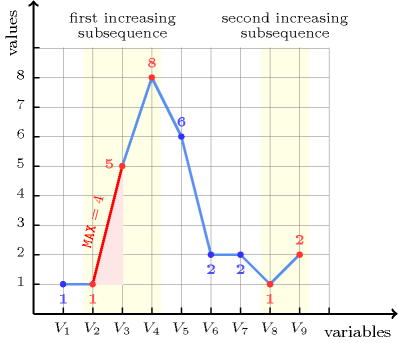Typical
 $\mathrm{𝙼𝙰𝚇}>0$ $\mathrm{𝙼𝙰𝚇}<$$\mathrm{𝚛𝚊𝚗𝚐𝚎}$$\left(\mathrm{𝚅𝙰𝚁𝙸𝙰𝙱𝙻𝙴𝚂}.\mathrm{𝚟𝚊𝚛}\right)-1$ $|\mathrm{𝚅𝙰𝚁𝙸𝙰𝙱𝙻𝙴𝚂}|>2$ $\mathrm{𝚛𝚊𝚗𝚐𝚎}$$\left(\mathrm{𝚅𝙰𝚁𝙸𝙰𝙱𝙻𝙴𝚂}.\mathrm{𝚟𝚊𝚛}\right)>2$
Symmetry

One and the same constant can be added to the $\mathrm{𝚟𝚊𝚛}$ attribute of all items of $\mathrm{𝚅𝙰𝚁𝙸𝙰𝙱𝙻𝙴𝚂}$.

Arg. properties

Functional dependency: $\mathrm{𝙼𝙰𝚇}$ determined by $\mathrm{𝚅𝙰𝚁𝙸𝙰𝙱𝙻𝙴𝚂}$.

Usage

Getting the maximum slope over the increasing sequences of time series.

Counting
 Length ($n$) 2 3 4 5 6 7 8 Solutions 9 64 625 7776 117649 2097152 43046721

Number of solutions for $\mathrm{𝚖𝚊𝚡}_\mathrm{𝚒𝚗𝚌𝚛𝚎𝚊𝚜𝚒𝚗𝚐}_\mathrm{𝚜𝚕𝚘𝚙𝚎}$: domains $0..n$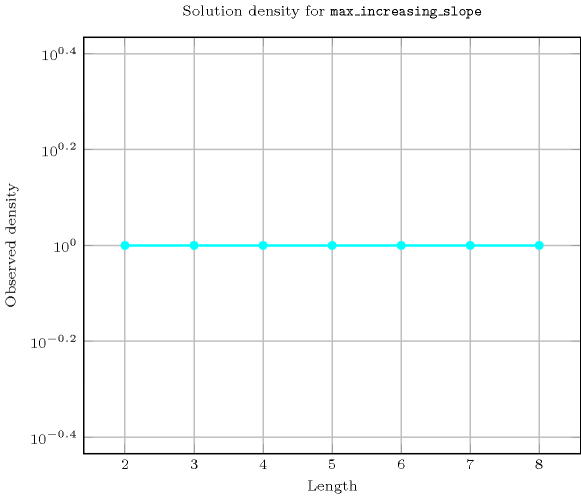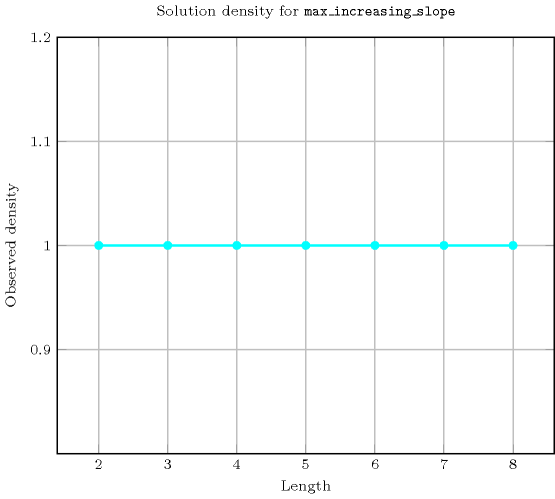Length ($n$)2345678
Total9646257776117649209715243046721
 Parameter value

062070252924343212870
12201511036682844220284405
21161881952192001833041721425
3-81422106290353801164847301
4--741584282664838408021350
5---846216844576329208124
6----117123530888654931
7-----1915206673834
8------3622481

Solution count for $\mathrm{𝚖𝚊𝚡}_\mathrm{𝚒𝚗𝚌𝚛𝚎𝚊𝚜𝚒𝚗𝚐}_\mathrm{𝚜𝚕𝚘𝚙𝚎}$: domains $0..n$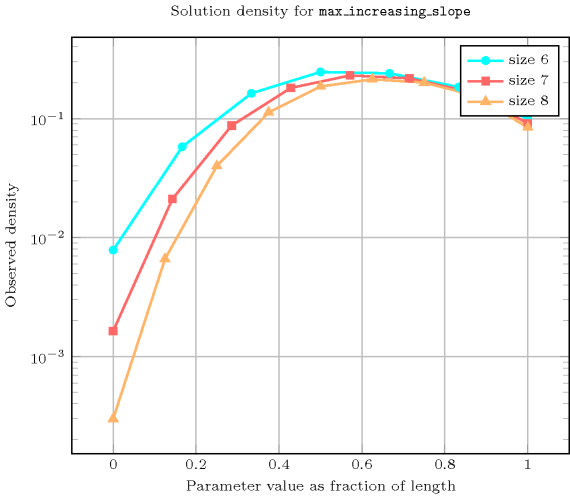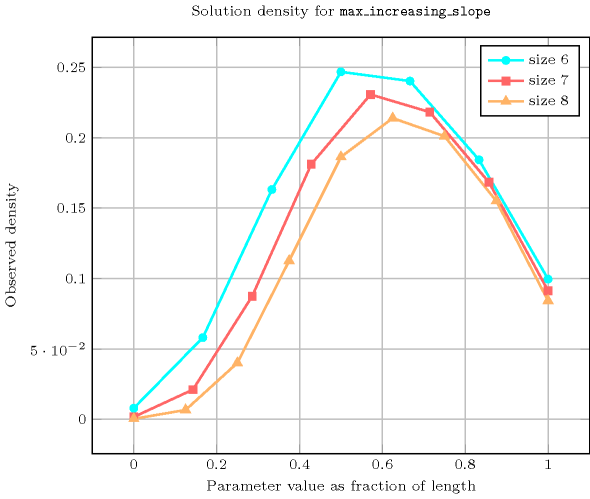Keywords
Cond. implications

$•$ $\mathrm{𝚖𝚊𝚡}_\mathrm{𝚒𝚗𝚌𝚛𝚎𝚊𝚜𝚒𝚗𝚐}_\mathrm{𝚜𝚕𝚘𝚙𝚎}\left(\mathrm{𝙼𝙰𝚇},\mathrm{𝚅𝙰𝚁𝙸𝙰𝙱𝙻𝙴𝚂}\right)$

with  $\mathrm{𝚛𝚊𝚗𝚐𝚎}$$\left(\mathrm{𝚅𝙰𝚁𝙸𝙰𝙱𝙻𝙴𝚂}.\mathrm{𝚟𝚊𝚛}\right)=\mathrm{𝙼𝙰𝚇}+1$

implies $\mathrm{𝚕𝚘𝚗𝚐𝚎𝚜𝚝}_\mathrm{𝚒𝚗𝚌𝚛𝚎𝚊𝚜𝚒𝚗𝚐}_\mathrm{𝚜𝚎𝚚𝚞𝚎𝚗𝚌𝚎}$$\left(𝙻,\mathrm{𝚅𝙰𝚁𝙸𝙰𝙱𝙻𝙴𝚂}\right)$

when  $\mathrm{𝚛𝚊𝚗𝚐𝚎}$$\left(\mathrm{𝚅𝙰𝚁𝙸𝙰𝙱𝙻𝙴𝚂}.\mathrm{𝚟𝚊𝚛}\right)=𝙻+1$.

$•$ $\mathrm{𝚖𝚊𝚡}_\mathrm{𝚒𝚗𝚌𝚛𝚎𝚊𝚜𝚒𝚗𝚐}_\mathrm{𝚜𝚕𝚘𝚙𝚎}\left(\mathrm{𝙼𝙰𝚇},\mathrm{𝚅𝙰𝚁𝙸𝙰𝙱𝙻𝙴𝚂}\right)$

with  $\mathrm{𝙼𝙰𝚇}=1$

implies $\mathrm{𝚖𝚒𝚗}_\mathrm{𝚒𝚗𝚌𝚛𝚎𝚊𝚜𝚒𝚗𝚐}_\mathrm{𝚜𝚕𝚘𝚙𝚎}$$\left(\mathrm{𝙼𝙸𝙽},\mathrm{𝚅𝙰𝚁𝙸𝙰𝙱𝙻𝙴𝚂}\right)$

when  $\mathrm{𝙼𝙸𝙽}=1$.

Automaton

Figure 5.241.2 depicts the automaton associated with the $\mathrm{𝚖𝚊𝚡}_\mathrm{𝚒𝚗𝚌𝚛𝚎𝚊𝚜𝚒𝚗𝚐}_\mathrm{𝚜𝚕𝚘𝚙𝚎}$ constraint. To each pair of consecutive variables $\left({\mathrm{𝚅𝙰𝚁}}_{i},{\mathrm{𝚅𝙰𝚁}}_{i+1}\right)$ of the collection $\mathrm{𝚅𝙰𝚁𝙸𝙰𝙱𝙻𝙴𝚂}$ corresponds a signature variable ${S}_{i}$. The following signature constraint links ${\mathrm{𝚅𝙰𝚁}}_{i}$, ${\mathrm{𝚅𝙰𝚁}}_{i+1}$ and ${S}_{i}$: $\left({\mathrm{𝚅𝙰𝚁}}_{i}\ge {\mathrm{𝚅𝙰𝚁}}_{i+1}⇔{S}_{i}=0\right)\wedge \left({\mathrm{𝚅𝙰𝚁}}_{i}<{\mathrm{𝚅𝙰𝚁}}_{i+1}⇔{S}_{i}=1\right)$.

##### Figure 5.241.2. Automaton for the $\mathrm{𝚖𝚊𝚡}_\mathrm{𝚒𝚗𝚌𝚛𝚎𝚊𝚜𝚒𝚗𝚐}_\mathrm{𝚜𝚕𝚘𝚙𝚎}$ constraint and its glue matrix (note that the reverse of $\mathrm{𝚖𝚊𝚡}_\mathrm{𝚒𝚗𝚌𝚛𝚎𝚊𝚜𝚒𝚗𝚐}_\mathrm{𝚜𝚕𝚘𝚙𝚎}$ is $\mathrm{𝚖𝚊𝚡}_\mathrm{𝚍𝚎𝚌𝚛𝚎𝚊𝚜𝚒𝚗𝚐}_\mathrm{𝚜𝚕𝚘𝚙𝚎}$)